Superposition with Dependent Sources

In the Superposition section we explored a problem-solving technique that allowed us to solve a linear circuit system by analyzing one source at a time. By turning all other sources off, we can look at each current or voltage source's contribution separately. These individual analyses became quite simple, and they can then be easily combined to regenerate the original solution.

With independent sources, it was easy to turn individual voltage and currents off. For independent voltage sources, we set their voltage to zero, effectively replacing them with a short circuit. For independent current sources, we set their voltages to zero, effectively replacing them with an open circuit.

How should we handle dependent sources in the context of superposition?

The answer is found if we go back to the math of linear systems of equations.

The dependent source equations we created for linear VCVS, VCCS, CCVS, and CCCS sources all have one feature in common: unlike independent sources, they have zero constant term in their equations:

\begin{align} V_X - V_Y - k_1 V_A + k_1 V_B & = 0 && \text{(for VCVS)} \\ i_2 - k_2 V_A + k_2 V_B & = 0 && \text{(for VCCS)} \\ V_X - V_Y - k_3 i_3 & = 0 && \text{(for CCVS)} \\ i_4 - k_4 i_5 & = 0 && \text{(for CCCS)} \end{align}

In the context of superposition, dependent sources look more like resistors, which simply relate various branch currents and node voltages. Their equations have no nonzero independent source terms on the right-hand side of the equation, so they do not effect superposition.

The result is that dependent sources do not get "turned off" during superposition. Instead, we still have to go through each independent source one at a time, but in each scenario, we still must consider any dependent sources to still be included without modification.

A Solved Example

Let's solve a circuit with two independent sources and one dependent source using superposition: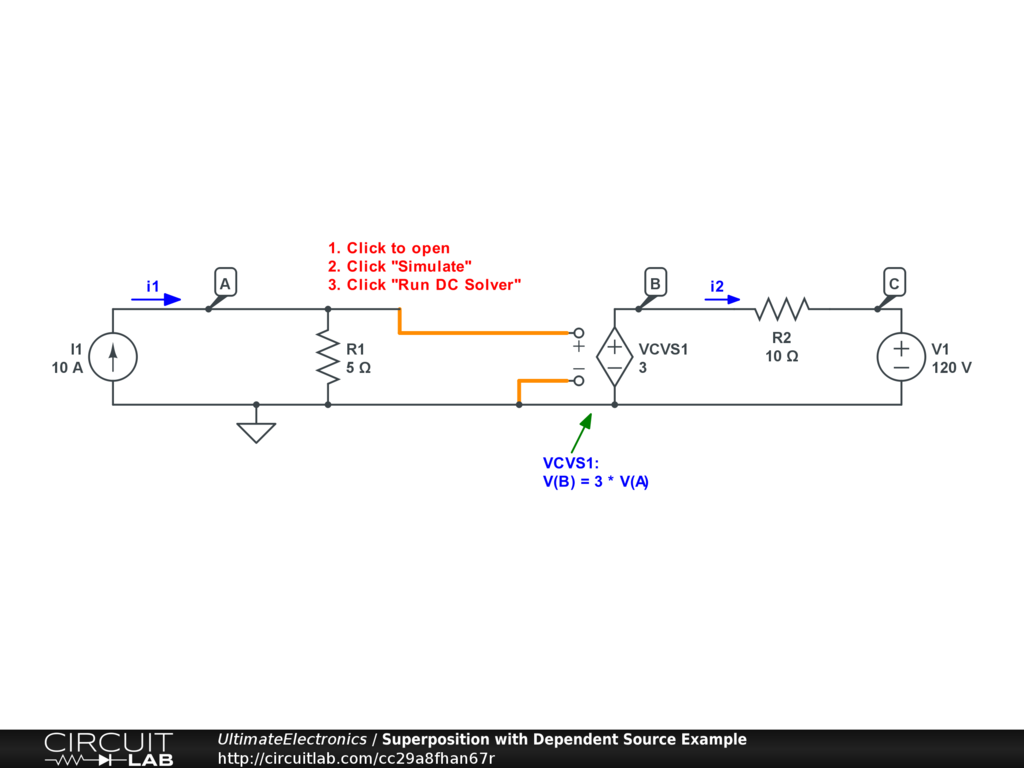Interactive Exercise Click to simulate the circuit above.

We've labeled this circuit with two branch currents $i_1, i_2$ and three non-ground node voltages $V_A, V_B, V_C$. Click to open the circuit, and try to solve the circuit by hand first. Then, click "Simulate," and "Run DC Solver" to check you answers.

As described in the Superposition section, this circuit has one solution when only independent source V1 is on, and another solution when only independent source I1 is on. In both cases, the dependent source VCVS1 will remain active.

For current source I1 only:

We can turn off V1 by setting it to zero, which means replacing it with a wire: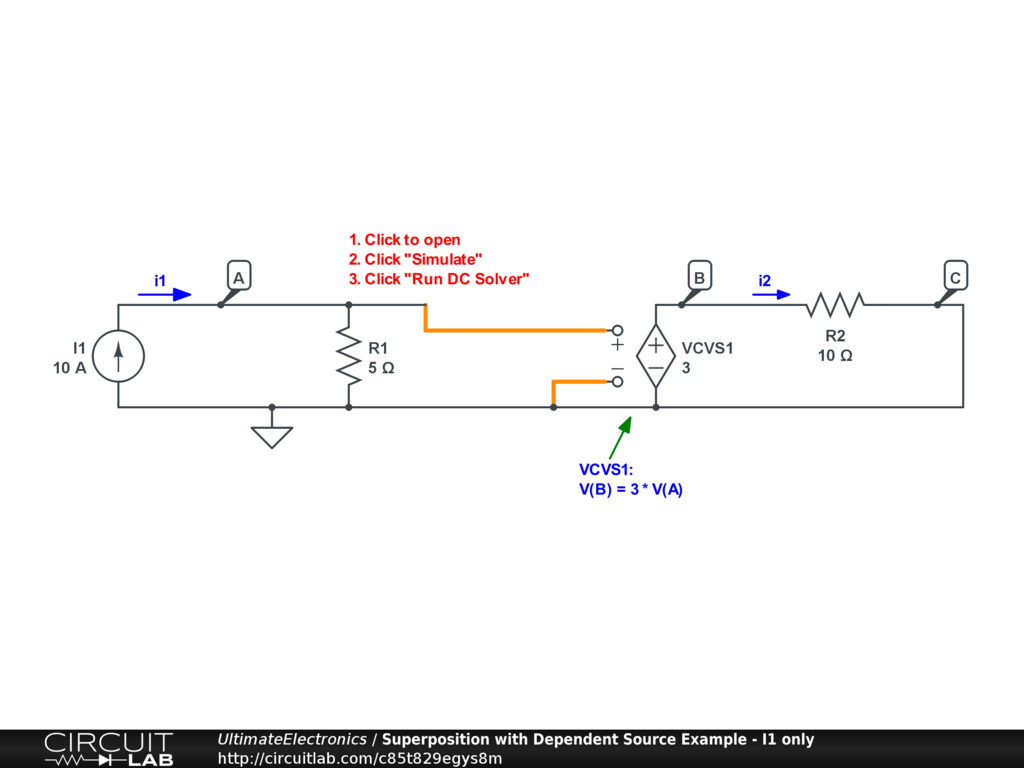Interactive Exercise Click to simulate the circuit above.

Now, the circuit is easy to solve by inspection. The current source I1 sets branch current $i_1=10$, which flows entirely through R1, forcing a voltage drop of $V_A = 5 i_1 = 50$. The dependent source VCVS1 multiplies this by 3 to get $V_B = 3 V_A = 150$. This voltage drives current through resistor R2 to now-grounded node C, so $V_C=0$ and $i_2 = \frac{150}{10} = 15$. The circuit solution vector for this superposition case is:

$$\begin{equation} \mathbf{x_{\text{I1}}} = \begin{bmatrix} i_1 \\ i_2 \\ V_A \\ V_B \\ V_C \end{bmatrix} = \begin{bmatrix} 10 \\ 15 \\ 50 \\ 150 \\ 0 \end{bmatrix} \end{equation}$$

For voltage source V1 only:

We can turn off I1 by setting it to zero, which means deleting it entirely and leaving an open circuit: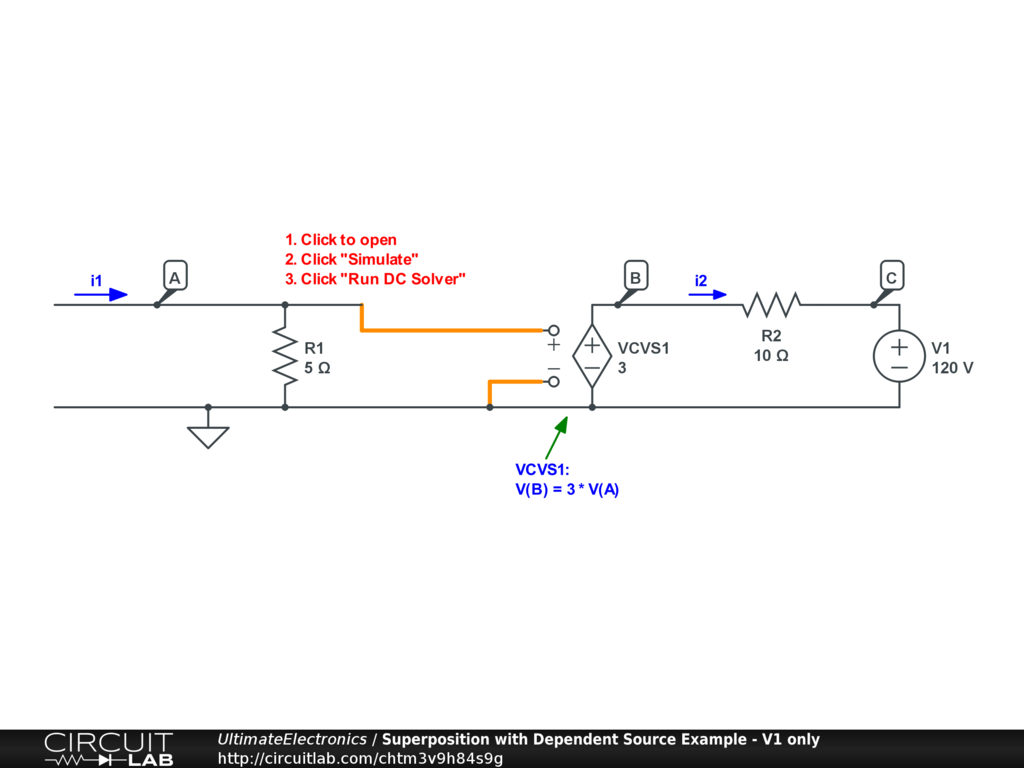Interactive Exercise Click to simulate the circuit above.

Again, this circuit is easy to solve by inspection. No current flows through branch $i_1 = 0$, so there is no voltage drop across R1 and $V_A = 0$. The dependent source VCVS1 multiplies this zero and itself outputs zero voltage, effectively making node B a ground node in this case $V_B = 0$. Now, current flows through R2 driven by $V_C = 120$ in a direction opposite to the arrow drawn for $i_2$, so $i_2 = -\frac{120}{10} = -12$. The circuit solution vector for this superposition case is:

$$\begin{equation} \mathbf{x_{\text{V1}}} = \begin{bmatrix} i_1 \\ i_2 \\ V_A \\ V_B \\ V_C \end{bmatrix} = \begin{bmatrix} 0 \\ -12 \\ 0 \\ 0 \\ 120 \end{bmatrix} \end{equation}$$

Combine the Parts:

Each of the two sub-problems were easy to solve, even with the dependent source. Now, we can use superposition to combine the individual solution vectors into the final circuit solution:

\begin{align} \mathbf{x} & = \mathbf{x_{\text{I1}}} + \mathbf{x_{\text{V1}}} \\ \mathbf{x} & = \begin{bmatrix} 10 \\ 15 \\ 50 \\ 150 \\ 0 \end{bmatrix} + \begin{bmatrix} 0 \\ -12 \\ 0 \\ 0 \\ 120 \end{bmatrix} \\ \mathbf{x} & = \begin{bmatrix} 10 \\ 3 \\ 50 \\ 150 \\ 120 \end{bmatrix} = \begin{bmatrix} i_1 \\ i_2 \\ V_A \\ V_B \\ V_C \end{bmatrix} \end{align}

This is the same solution we found when solving the entire circuit by hand or by simulation above.

Thevenin and Norton Equivalent Circuits with Dependent Sources

In the Thevenin Equivalent and Norton Equivalent Circuits we explored how to use superposition to find simplified equivalent circuits looking in any pair of nodes. Superposition was useful because we added a test current source connected to our nodes of interest. It was then easy to turn off all independent sources, at which point the circuit could often be collapsed by resistor network reduction.

However, when our circuit has dependent sources, we usually can't so easily reduce resistor networks.

Using Thevenin equivalent circuits is extremely common in analyzing nonlinear circuits like transistor amplifiers, where we create a linearized model of a nonlinear circuit. For example, this linear circuit has relevance to some transistor amplifiers we'll study later: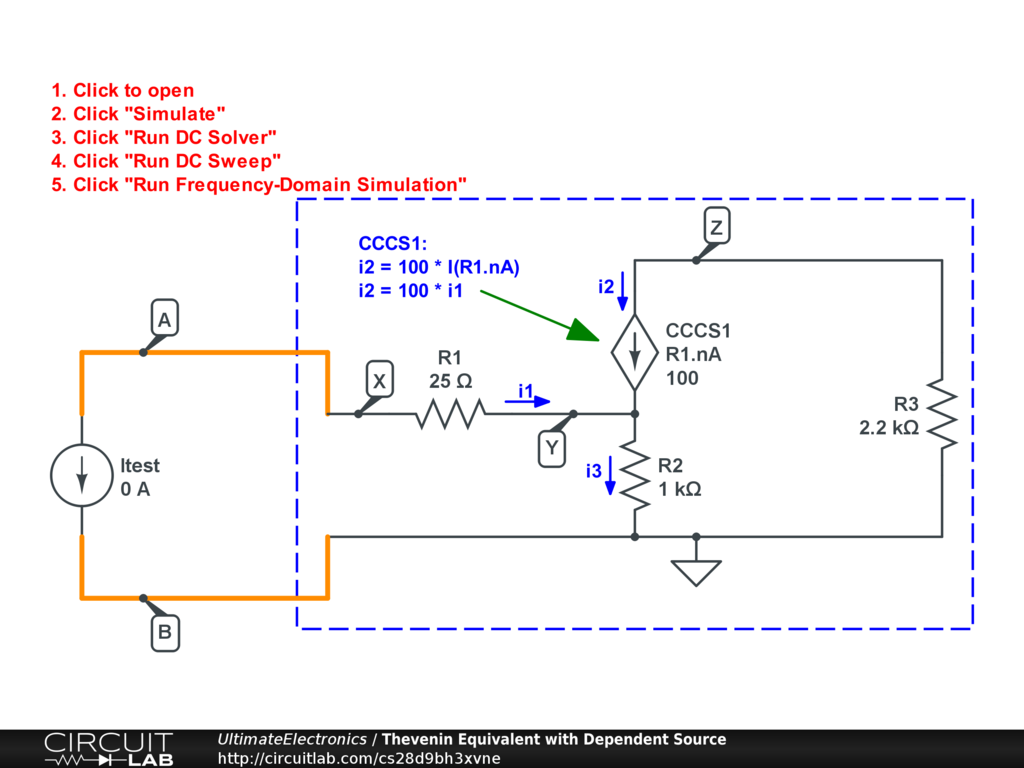Interactive Exercise Click to simulate the circuit above.

Our black box has a three resistors and a current-controlled current source. This acts like a simple model of a Bipolar Junction Transistor amplifier: a small base current $i_1$ is amplified 100X to become a collector current $i_2$. The total current $i_3 = i_1 + i_2$ flows out the emitter.

What is the Thevenin equivalent circuit looking into nodes X and ground of this circuit? Is it something you can easily see from the values of the components as shown?

Despite the added complexity in this example, the same computational technique shown in the Finding the Thevenin Equivalent Circuit by Simulation section applies. The open-circuit voltage is 0 because there are no nonzero independent sources. The Frequency Domain simulation of $\text{REAL(V(A)} - \text{V(B))}$ reveals the sensitivity of the voltage difference to a chance in test current, which is exactly the meaning of the Thevenin equivalent impedance. In this example, running the Frequency Domain simulation shows a flat line at -101025, indicating that for every 1 unit that Itest goes increases, the voltage difference $V_{A B}$ decreases by 101025. This is just like a resistor of $R_{\text{eq}} = 101025 \ \Omega$, with zero open circuit voltage $V_{\text{eq}} = 0$: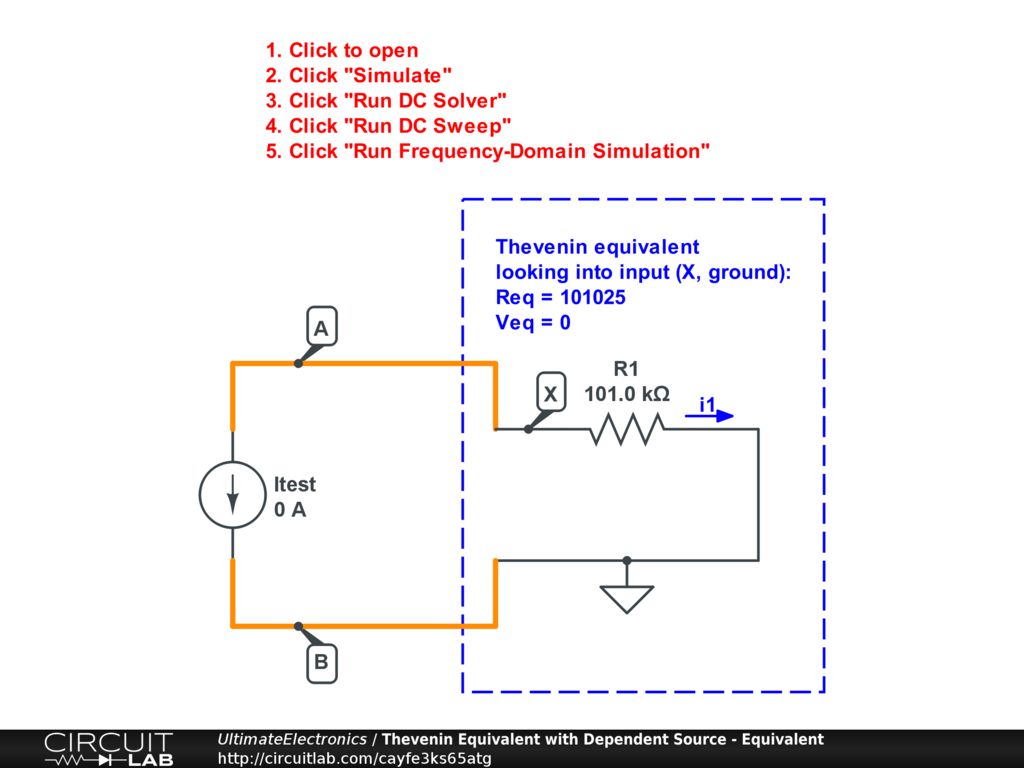Interactive Exercise You can run the Frequency Domain simulation, or the DC Sweep simulation of this Thevenin equivalent circuit and verify that -- from the perspective of the two selected terminals -- this one-resistor equivalent circuit behaves identically to the original.

The big idea is that from the perspective of one pair of terminals (for example, the input to a transistor amplifier), a complicated behavior may emerge from dependent sources that is not necessarily obvious at first glance. In this particular case, the high current gain of 100 means that the input impedance of this amplifier is very high, which can be a good thing if we're interested in maximum voltage signal transfer.

Solving by Hand

While the simulator made it extremely easy to find the Thevenin equivalent circuit, we can also solve a simple case like this by hand. For the same circuit as above:We're solving for an equivalent resistance defined as:

$$R_{\text{eq}} = - \frac {d V_{A B}} {d I_{\text{test}}} = \frac {d V_X} {d i_1}$$

since $I_{\text{test}} = - i_1$, $V_B = 0$, and $V_A = V_X$.

Can we write $V_X$ in terms of $i_1$? Yes, as from Ohm's Law on resistor R1 we have:

$$V_X - V_Y = 25 \cdot i_1$$

Similarly, for resistor R2 we can write:

$$V_Y = 1000 \cdot i_3$$

The trick is that $i_3 = i_1 + i_2$ from Kirchhoff's Current Law at node Y, and the controlled source CCCS1 defines $i_2 = 100 i_1$, so overall:

$$i_3 = i_1 + 100 \cdot i_1 = 101 \cdot i_1$$

When we substitute this back into the two Ohm's Law equations for R1 and R2, we find:

\begin{align} V_X & = V_Y + 25 \cdot i_1 \\ V_X & = (1000 \cdot i_3) + 25 \cdot i_1 \\ V_X & = (1000 \cdot 101 i_1) + 25 \cdot i_1 \\ V_X & = 101025 \cdot i_1 \end{align}

Now that we have a single equation relating $V_X$ to $i_1$, the value of the resistance $R_{\text{eq}} = 101025 \ \Omega$ is clear.

Practice solving this by hand. Current and voltage multiplication effects due to dependent sources are a tremendously important component of understanding most amplifier circuits.

In this circuit, the intuitive understanding is that a tiny current pushed into $i_1$ causes a ~100X larger current to flow through the resistor R2. The effective input resistance measures the change in voltage for a small change in current, and that change is dominated by this product term of about $100 \ \text{k}\Omega$, even though that resistance is far bigger than any of the resistors placed explicitly within the circuit.

Open the circuit and run the DC Sweep simulation to see both that a tiny current $i_1$ causes a substantial voltage change $V_{A B}$, and further that there's voltage amplification by a factor of approximately $- \frac {R_3} {R_2} = -2.2$ at the output $V_Z$.

What's Next

In the next section, Switches, we'll talk about buttons and switches that allow non-permanent connections within a circuit.